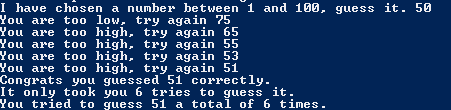# Assignemnt #66

## Code

```///colin hinton
///5th period
///hilo
///Hilo.java
///11/5/2015

import java.util.Scanner;
import java.util.Random;

class Hilo
{
public static void main (String [] args)
{
Scanner keyboard = new Scanner(System.in);
int guess;
int total = 0;

System.out.print("I have chosen a number between 1 and 100, guess it. ");
guess = keyboard.nextInt();
total++;

Random r = new Random();

int x = (1 + r.nextInt(100));

while (x > guess && total < 7)
{
System.out.print("You are too low, try again ");
guess = keyboard.nextInt();
total++;
}
while  ( x < guess && total < 7)
{
System.out.print("You are too high, try again ");
guess = keyboard.nextInt();
total++;
}
if (guess == x )
{
System.out.println("Congrats you guessed " + guess + " correctly.");
System.out.println("It only took you " + total + " tries to guess it.");
}

System.out.println("You tried to guess " + x + " a total of " + total + " times.");

}
}

```

### Picture of the output